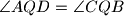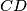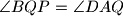### IMO Shortlist 2007 problem G3

Kvaliteta:
Avg: 0.0
Težina:
Avg: 7.0
The diagonals of a trapezoid$ABCD$ intersect at point$P$. Point$Q$ lies between the parallel lines$BC$ and$AD$ such that$\angle AQD = \angle CQB$, and line$CD$ separates points$P$ and$Q$. Prove that$\angle BQP = \angle DAQ$.

Author: unknown author, Ukraine
Source: Međunarodna matematička olimpijada, shortlist 2007